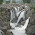### Difference between foo(void) and foo()

Source: http://stackoverflow.com/

Problem:
Consider these two function definitions
``````void foo(){ ... }
void foo(void){ ..... }``````
What is the difference between these two functions?

Hint: The answer depends whether this is C code or C++ code.

1.The difference is this,
foo(void) is the same in C/C++ a function with no arguments.
foo() in c++ also means the same as above.
foo() in c means that we there is not specified about the arguments of function foo() and about the type.

2.Taken from the book :The Complete Reference C++ - Page 626 - Edition #4

---
int f();
In C this means that the function f may or Might not have any parameters !!
--
---
In C++ "Super Set Of C"
int f() is same as int f(void)
because void parameter list is optional in C++
But programmers put void inside () making it clear that no parameters are accepted by function !!

Example ::

C Code :
#include
void f()
{
puts("hello there");
}
int main()
{
f(66);
}
----

The above code will compile without error and print "hello there"

C++ example:

#include
using namespace std;
void f( )
{
cout<<"from f"<<endl;
}
int main()
{
f(3);
return 0;
}

The above code will say that(compiler)
too many arguments supplied !!
---